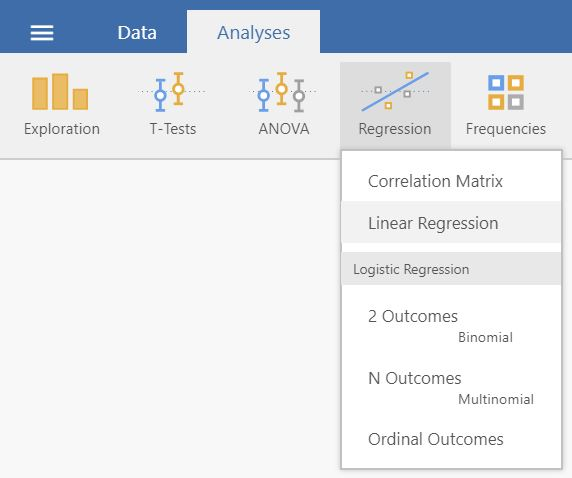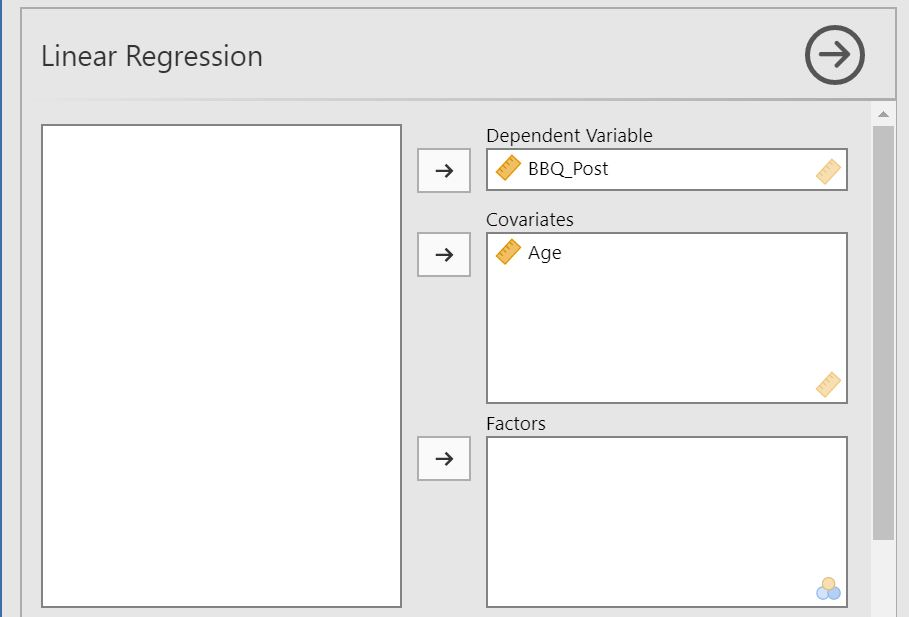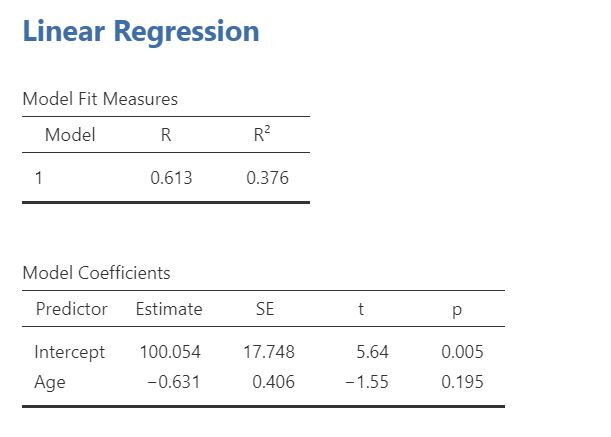# 11 Linear Regression

#### How to perform a linear regression in jamovi:

1. You need one predictor variable and one continuous outcome variable. Make sure that the measurement levels are set9.
A correct setup should look similar to this:2. Select `Analyses` -> `Regression` -> `Linear Regression`.3. Drag and drop your outcome variable to Dependent Variable and your predictor variable to Covariates if it is continuous or to Factors if it is categorical.4. The result is shown in the right panel:1. This is demonstrated in section 2.